• 关于随机森林的简介和应用理论，请阅读之前分享的文章：一文读懂随机森林在微生态中的应用关于随机森林进行分类的入门实战，请阅读之前分享的《RandomForest：随机森林预测生物标记bio...


关于随机森林的简介和应用理论，请阅读之前分享的文章：一文读懂随机森林在微生态中的应用关于随机森林进行分类的入门实战，请阅读之前分享的《RandomForest：随机森林预测生物标记biomarker——分类》，大家可以学习此文，实现分组挖掘两组或多组的特异Features，也可以展示特征的贡献度，获得分类评估的准确度，以及使用新数据进行预测，无监督的随机森林等基础技能。今天我们讲使用randomForest实现回归分析的实战代码。回归的应用主要包括时间序列预测模式，如预测股票、尸体死亡时间等。本节不需要先验知识可也直接学习使用。RandomForest安装与加载# 安装
install.packages("randomForest")
# 加载
library(randomForest)回归Classification先了解一下输入数据格式，方便准备使用R内置按天记录的空气质量数据data(airquality)
head(airquality)数据包括157天中，臭氧、太阳强度、风和温度，部分有缺失。前4列属性数据，后2列时间月和日为分组数据。  Ozone Solar.R Wind Temp Month Day
1    41     190  7.4   67     5   1
2    36     118  8.0   72     5   2
3    12     149 12.6   74     5   3
4    18     313 11.5   62     5   4
5    NA      NA 14.3   56     5   5
6    28      NA 14.9   66     5   6设置随机数种子保证结果可重复set.seed(315)随机森林回归臭氧与其它所有属性ozone.rf= randomForest(Ozone ~ ., data=airquality, mtry=3,
importance=TRUE, na.action=na.omit)
print(ozone.rf)结果如下：包括分析的命令，分析类型，树数量，重要的变量(Feature)个数，平均残差平方，解析率。    Call:
randomForest(formula = Ozone ~ ., data = airquality, mtry = 3,      importance = TRUE, na.action = na.omit)
Type of random forest: regression
Number of trees: 500
No. of variables tried at each split: 3

Mean of squared residuals: 304.4269
% Var explained: 72.26查看每个变量的分类贡献度，显示仅保留两位小数可读性更好round(importance(ozone.rf), 2)

%IncMSE IncNodePurity
Solar.R   10.40      10833.08
Wind      23.55      43838.66
Temp      47.13      53731.95
Month      2.04       1504.72
Day        0.91       6306.42结果为每个相关变量对应两列值。%IncMSE是Increased in mean squared error (%)，直译为增长的错误率平方均值，即去除该变量后，对目标预测的准确度下降的低，可理解为对目标变量预测准确的贡献度。IncNodePurity是Increased node purity，是另一种评估的方法。这里我们只关注%IncMSE就够了。varImpPlot(ozone.rf)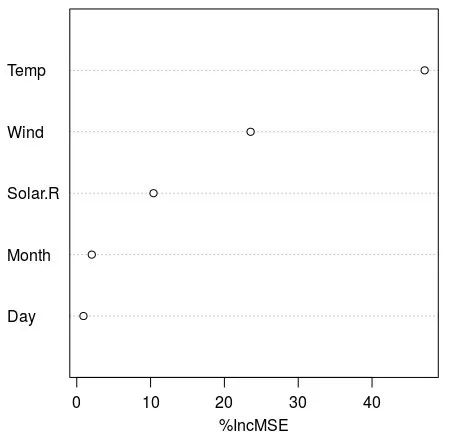交叉验证cross-validation# 先清空NA的样本，验证不允许有NA
airquality = na.omit(airquality)
myairquality= cbind(airquality[1:6], matrix(runif(96 * nrow(airquality)), nrow(airquality), 96))
# 交驻验证添加了随机数的训练集，分组，交叉验证的次数
result= rfcv(myairquality, airquality$Ozone, cv.fold=3) # 绘制错误率曲线，观查错误率与使用Markers数量的变化 with(result, plot(n.var, error.cv, log="x", type="o", lwd=2))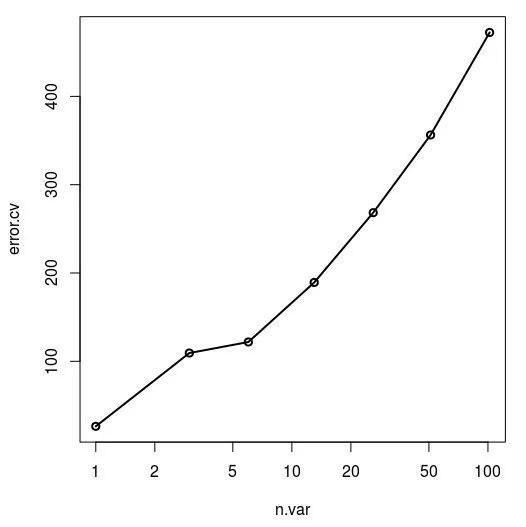我们看到一个现象，不是feature越多越好，无关的feature如果多了，反而错误率上升，会影响预测的准确度。# 使用replicate进行多次交叉验证，可选 result= replicate(5, rfcv(myairquality, airquality$Ozone), simplify=FALSE)
error.cv= sapply(result, "[[", "error.cv")
matplot(result[]\$n.var, cbind(rowMeans(error.cv), error.cv), type="l",
lwd=c(2, rep(1, ncol(error.cv))), col=1, lty=1, log="x",
xlab="Number of variables", ylab="CV Error")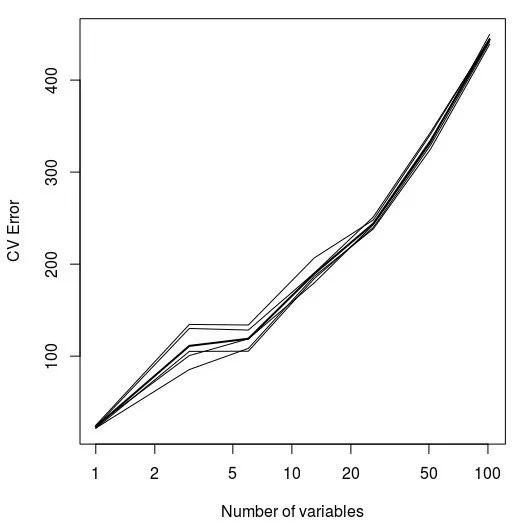多次验证结果类型，更能说明结果的可信度。严谨总没有坏处，好的结果都是多角度证明的。大家学习随机森林的分类、和回归。将来有时间，将带大家上手重复一些高水平文章中的分析，结合具体生物学问题会更有意思。猜你喜欢10000+：菌群分析 宝宝与猫狗 梅毒狂想曲 提DNA发Nature Cell专刊 肠道指挥大脑系列教程：微生物组入门 Biostar 微生物组  宏基因组专业技能：学术图表 高分文章 生信宝典 不可或缺的人一文读懂：宏基因组 寄生虫益处 进化树必备技能：提问 搜索  Endnote文献阅读 热心肠 SemanticScholar Geenmedical扩增子分析：图表解读 分析流程 统计绘图16S功能预测   PICRUSt  FAPROTAX  Bugbase Tax4Fun在线工具：16S预测培养基 生信绘图科研经验：云笔记  云协作 公众号编程模板: Shell  R Perl生物科普:  肠道细菌 人体上的生命 生命大跃进  细胞暗战 人体奥秘  写在后面为鼓励读者交流、快速解决科研困难，我们建立了“宏基因组”专业讨论群，目前己有国内外5000+ 一线科研人员加入。参与讨论，获得专业解答，欢迎分享此文至朋友圈，并扫码加主编好友带你入群，务必备注“姓名-单位-研究方向-职称/年级”。PI请明示身份，另有海内外微生物相关PI群供大佬合作交流。技术问题寻求帮助，首先阅读《如何优雅的提问》学习解决问题思路，仍未解决群内讨论，问题不私聊，帮助同行。学习16S扩增子、宏基因组科研思路和分析实战，关注“宏基因组”点击阅读原文，跳转最新文章目录阅读

展开全文• 既然随机森林预测结果是所有决策树结果的平均值。而决策树的预测结果是决策树叶子结点对应数据的平均值。那么预测结果不可能超过训练数据的最大值。但是实际运行过程中不是这样的，是不是我理解错了？...人工智能 机器学习
• 思路个前面的两篇一致（xg,lgbm），只是换了一个数据集，感兴趣的小伙伴可以将这个代码换成之前的数据集，这里我是预测为了7天的数据，利用一年的数据。另外采用的调参方式也进行了改变，这次不是hyperopt，也是grid...
   思路个前面的两篇一致（xg,lgbm），只是换了一个数据集，感兴趣的小伙伴可以将这个代码换成之前的数据集，这里我是预测为了7天的数据，利用一年的数据。另外采用的调参方式也进行了改变，这次不是hyperopt，也是gridsearch

import pandas as pd
import gc
from numpy import nan
from numpy import isnan
from pandas import read_csv
from pandas import to_numeric

from sklearn.metrics import r2_score
import lightgbm as lgb
# multivariate multi-step encoder-decoder lstm
from math import sqrt
from numpy import split
from numpy import array
from pandas import read_csv
from sklearn.metrics import mean_squared_error
from matplotlib import pyplot

from sklearn.metrics import r2_score
from sklearn.preprocessing import MinMaxScaler
from sklearn.preprocessing import LabelEncoder
from numpy.random import seed
import numpy as np
import xgboost as xgb
import pandas as pd
#from sklearn.metrics import roc_auc_score
from sklearn.metrics import explained_variance_score
import matplotlib.pyplot as plt

from hyperopt import STATUS_OK,STATUS_RUNNING, fmin, hp, tpe,space_eval

from sklearn.model_selection import train_test_split
from sklearn.metrics import roc_auc_score
SEED = 314159265
VALID_SIZE = 0.25

sheet = pd.read_excel('F:\\123123.xlsx',sheet_name= [0,1,2,3],header=0, index_col=0)
#dataset=sheet.astype('float64')
#values=dataset.values
print(sheet)
data=sheet
ans=sheet.copy()
print(data.head())

# convert timeserise to supervised-learn
def to_supervised(data):
x = data.iloc[0:360,:].values
y = data.iloc[7:367,3].values
return x, y
data_x,data_y=to_supervised(sheet)
print(data_x.shape)
print(data_y.shape)
train_x,test_x=data_x[0:300],data_x[300:367]
train_y,test_y=data_y[0:300],data_y[300:367]

print(test_x.shape)
print(train_x.shape)
print(test_y.shape)
print(train_y.shape)
from sklearn.model_selection import GridSearchCV
from sklearn.ensemble import RandomForestRegressor

rfgs_parameters = {
'n_estimators': [n for n in range(30, 50)],
'max_depth'   : [n for n in range(2, 6)],
'max_features': [n for n in range(2, 6)],
"min_samples_split": [n for n in range(2, 4)],
"min_samples_leaf": [n for n in range(2, 4)],
"bootstrap": [True,False]
}

rfr_cv = GridSearchCV(RandomForestRegressor(), rfgs_parameters, cv=8, scoring= 'neg_mean_squared_log_error')
rfr_cv.fit(train_x, train_y)
print("RFR GridSearch score: "+str(rfr_cv.best_score_))
print("RFR GridSearch params: ")
print(rfr_cv.best_params_)
prediction1 = rfr_cv.best_estimator_.predict(test_x)
print(prediction1)
print(r2_score(test_y,prediction1))
prediction2 = rfr_cv.best_estimator_.predict(test_x[364:368,:])
print(prediction2)
"""

print("---------优化完成----------")
##训练模型
print(best_params)
print("---------正式训练模型----------")
watchlist = [(dtrain, 'train'), (dvalid, 'eval')]
model_gbm = xgb.train(best_params, dtrain, 1000, evals=watchlist,early_stopping_rounds=1000,verbose_eval=True)
print("---------正式预测模型----------")
print("Predict test set...")
test_prediction = model_gbm.predict(xgb.DMatrix(test_x), ntree_limit=model_gbm.best_iteration+1)
print("---------预测完成----------")
print(test_prediction)

test_prediction = model_gbm.predict(xgb.DMatrix(test_x[364:368,:]), ntree_limit=model_gbm.best_iteration+1)
print("---------预测完成----------")
print(test_prediction)
"""


展开全文• 对于零售行业来说，预测几乎是商业智能（BI）研究的终极问题，单纯从机器学习的角度来说，做到精准预测很容易，但是结合业务提高企业利润却很难。预测精确性是核心痛点。 业务挑战 扎对，将产品粗略分为：基本款...
原文链接：http://tecdat.cn/?p=1130

原文出处：拓端数据部落公众号如今DT（数据技术）时代，数据变得越来越重要，其核心应用“预测”也成为互联网行业以及产业变革的重要力量。对于零售行业来说，预测几乎是商业智能（BI）研究的终极问题，单纯从机器学习的角度来说，做到精准预测很容易，但是结合业务提高企业利润却很难。预测精确性是核心痛点。

视频：机器学习助推精准销量预测 part1

业务挑战

扎对，将产品粗略分为：基本款和时装。对于基本款，每年都没什么大变化，国际流行的影响也不大，那么可以进行长计划生产。对于时装，决定潮流走向的决策权不在某个区域，一个地方的买手们也没有成长到可以准确预判国际流行趋势，所以需要结合不同区域的各种因素，进行预测。对应的，在新货构成中，销量预测策略为：基本款计划生产，时尚款机动调整。解决方案

任务/目标

根据服装零售业务营销要求，运用多种数据源分析实现精准销量预测。

数据源准备

沙子进来沙子出，金子进来金子出。无数据或数据质量低，会影响模型预测效果。在建立的一个合理的模型之前，对数据要进行收集，搜集除已有销量数据之外的额外信息（比如天气，地点，节假日信息等），再在搜集的数据基础上进行预处理。

有了数据，但是有一部分特征是算法不能直接处理的，还有一部分数据是算法不能直接利用的。

特征转换

把不能处理的特征做一些转换，处理成算法容易处理的干净特征举例如下：

销售日期。就时间属性本身来说，对模型来说不具有任何意义，需要把日期转变成到年份，月份，日，周伪变量。

产品特征。从产品信息表里面可以得到款式，颜色，质地以及这款产品是否是限量版等。然而并没有这些变量。这就需要我们从产品名字抽取这款产品的上述特征。

以上例举的只是部分特征。

构造

以上说明了如何抽取相关特征，我们大致有如下训练样本（只列举部分特征）。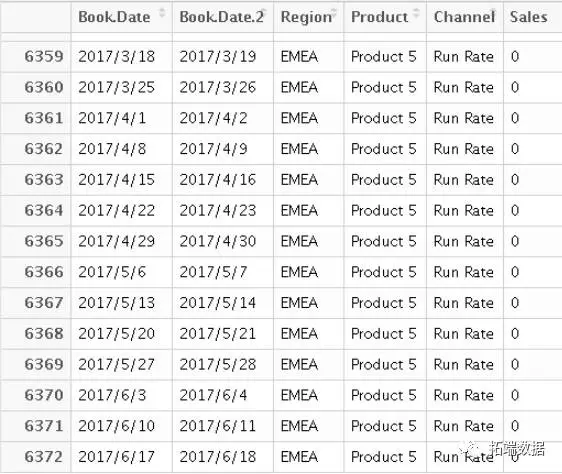划分训练集和测试集

考虑到最终模型会预测将来的某时间段的销量，为了更真实的测试模型效果，以时间来切分训练集和测试集。具体做法如下：假设我们有2014-02-01~2017-06- 17的销量相关数据。以2014-02-01~2016-03-19的销量数据作为训练，2016-03-20~2017-06-17的数据作为测试。

建模

ARIMA，一般应用在股票和电商销量领域

ARIMA模型是指将非平稳时间序列转化为平稳时间序列，然后将结果变量做自回归（AR）和自平移（MA）。

随机森林

用随机的方式建立一个森林，森林由很多决策树组成，随机森林的每一棵决策树之间是没有关联的。在得到森林之后，当有一个新的输入样本进入的时候，就让森林中的每一棵决策树分别进行一下判断，看看这个样本应该属于哪一类（对于分类算法），然后看看哪一类被选择最多，就预测这个样本为那一类。

支持向量回归（SVR）

SVR最本质与SVM类似，都有一个缘，只不过SVM的保证金是把两种类型分开，而SVR的保证金是指里面的数据会不会对回归有帮助。

模型优化

1.上线之前的优化：特征提取，样本抽样，参数调参。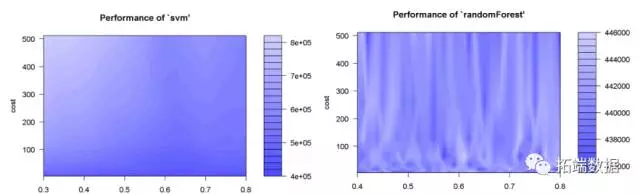2.上线之后的迭代，根据实际的A / B测试和业务人员的建议改进模型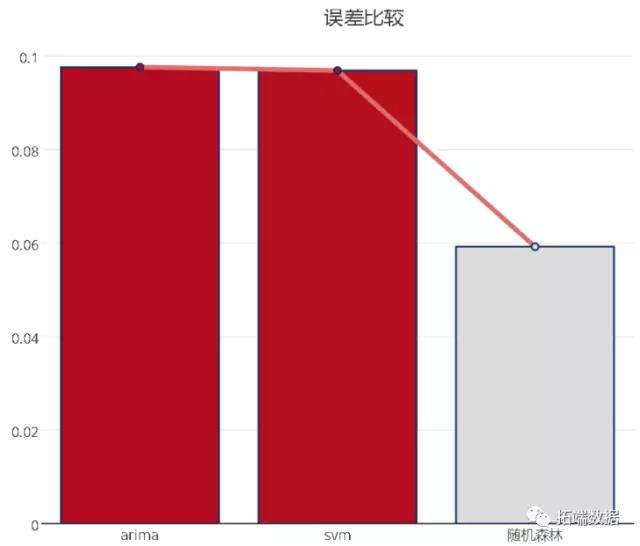从上图可以看出，在此案例中，支持向量机和随机森林算法模型的预测误差最小，运用3种方法预测某商品的销量，其可视化图形如下：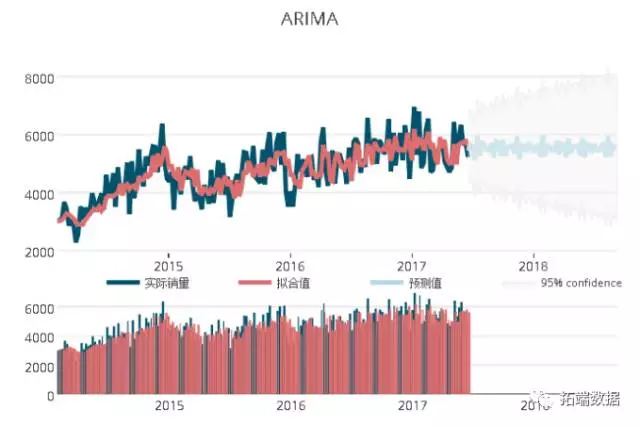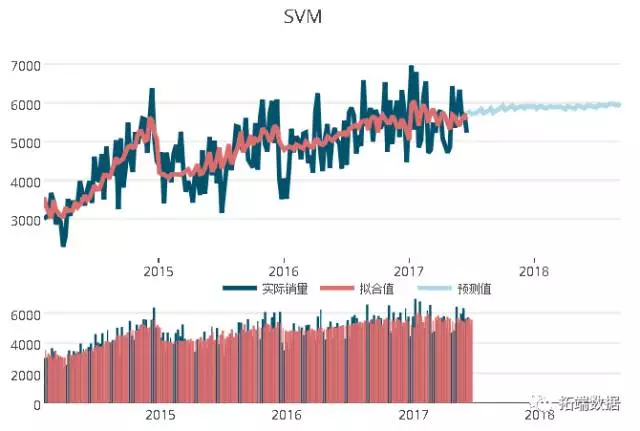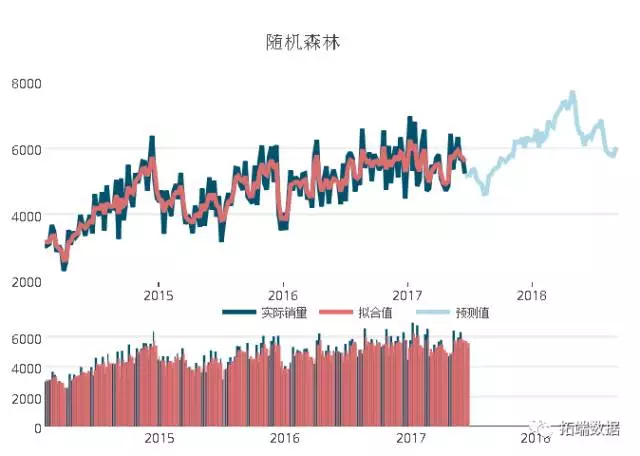可以看出，销量的预测值的趋势已经基本与真实销量趋势保持一致，但是在预测期较长的区间段，其预测值之间的差别较大。

评估效果不能只看销量，要综合考虑，需要参考业务对接，预测精度，模型可解释性和产业链整体能力等因素综合考虑;不能简单作为企业利润增加的唯一标准我们的经验是，预测结果仅作为参考一个权重值，还需要专家意见，按照一定的权重来计算。

展望

除了以上列举的一些方法，我们已经在尝试更复杂的销售预测模型，如HMM，深度学习（Long Short-Term Memory网络，卷积神经网络（CNN））等;同时需要考虑到模型的可解释性，可落地性和可扩展性，避免“黑箱”预测 ;还在尝试采用混合的机器学习模型，比如GLM + SVR，ARIMA + NNET等。

销售预测几乎是商业智能研究的终极问题，即便通过机器学习算法模型能够提高测试集的预测精度，但是对于未来数据集的预测，想做到精准预测以使企业利润最大化，还需要考虑机器学习模型之外的企业本身因素。比如，企业的整体供应链能力等，如何将企业因素加入到机器学习模型之中，是未来预销售预测的一个难点与方向。因此，要想解决销售预测终极问题还有一段路要走。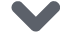相关文章：

使用R语言进行时间序列（arima，指数平滑）分析

在Python中使用LSTM和PyTorch进行时间序列预测

R语言k-Shape时间序列聚类方法对股票价格时间序列聚类

用广义加性模型GAM进行时间序列分析

R语言时间序列和ARIMA模型预测拖拉机销售的制造案例

在r语言中使用GAM（广义相加模型）进行电力负荷时间

R语言从经济时间序列中用HP滤波器，小波滤波和经验..

R语言COPULA和金融时间序列案例

用Prophet在Python中进行时间序列预测

R语言估计时变VAR模型时间序列的实证研究分析案例

Python中利用长短期记忆模型LSTM进行时间序列预测分析

Jenkins），SARIMA和ARIMAX模型用于预测时间序列数据

R语言对用电负荷时间序列数据进行K-medoids聚类建模和...

R语言多元Copula GARCH 模型时间序列预测

展开全文• 【原创】基于ARIMA、SVM、随机森林销售的时间序列预测数据分析报告论文（代码数据）.docx
• 时间序列数据是一种典型的数据，时间序列预测方法比较多。比如ARIMA模型、Prophet模型、指数平均法、滑动平均法等等。... 本文采用机器学习算法，如线性回归、随机森林等，完成时间序列预测预测效果也比较好。
文章目录1.简述2.数据集3.特征介绍（精髓）4.预测模型5.预测结果6.参考
1.简述

时间序列数据是一种典型的数据，时间序列预测方法比较多。比如ARIMA模型、Prophet模型、指数平均法、滑动平均法等等。
本文采用机器学习算法，如线性回归、随机森林等，完成时间序列预测，预测效果也比较好。

2.数据集
本文对应的数据集格式如下：

time
value

2018-09-01 00:00
3221

2018-09-01 01:00
5515

2018-09-01 02:00
9971

…
…

2018-09-05 01:00
4416

…
…

如1小时粒度数据。根据历史数据，对未来一段时间数据进行预测。
3.特征介绍（精髓）
由于是单变量数据，特征主要包括两部分：平移特征和时间特征。
平移特征是指，将value向前已经平移操作shift。
时间特征指，每分钟均值、每小时均值、每工作日均值、是否节假日等。
平移特征：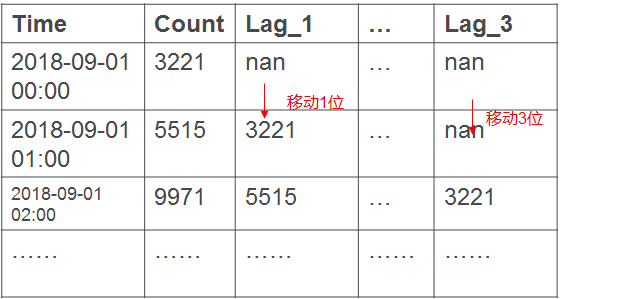时间特征：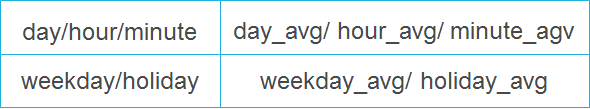当然，读者可以自行思考，是否有其他特征，也欢迎留言，大家一起探讨。
4.预测模型
import pandas as pd
import matplotlib.pylab as plt
import numpy as np
from sklearn.model_selection import cross_val_score
from sklearn.model_selection import TimeSeriesSplit
from sklearn.preprocessing import StandardScaler
from sklearn.linear_model import LassoCV, RidgeCV, LinearRegression
from sklearn.svm import SVR
from sklearn.ensemble import RandomForestRegressor
from sklearn.model_selection import GridSearchCV

# error计算误差
def mean_absolute_error(y_true, y_pred):
return np.mean(np.abs((y_true - y_pred) / y_true)) * 100

# data split分割数据，训练集、测试集、预测集
def timeseries_split(x, y, test_size, pred_size):
index_test = int(len(x) * (1 - test_size))
x_train = x.iloc[:index_test]
y_train = y.iloc[:index_test]
x_test = x.iloc[index_test:len(x) - pred_size]
y_test = y.iloc[index_test:len(x) - pred_size]
x_pred = x.iloc[-pred_size:]
y_pred = y.iloc[-pred_size:]
return x_train, y_train, x_test, y_test, x_pred, y_pred

# calculate mean计算均值特征
def cal_mean(data, x_feature, y_feature):
return dict(data.groupby(x_feature)[y_feature].mean())#利用分组，计算均值特征

# make feature计算平移特征
def build_feature(data, lag_start, lag_end, test_size, target_encoding=False, num_day_pred=1):#target_encoding是否要开启均值特征，num_day_pred预测多少天
# build future data with 0
last_date = data["time"].max()
# 预测点个数，由数据粒度决定
pred_points = int(num_day_pred * 24)  # 1h粒度，1day = 24个点
pred_date = pd.date_range(start=last_date, periods=pred_points + 1, freq="1h")
pred_date = pred_date[pred_date > last_date]  # 排除掉last_date(非预测)， preiods = pred_points +1,也是因为last_date为非预测point，所以后延1个point
future_data = pd.DataFrame({"time": pred_date, "y": np.zeros(len(pred_date))})#先将预测时间段的value设置为0，然后在利用shift和均值等构件特征，做预测
# concat future data and last data
df = pd.concat([data, future_data])
df.set_index("time", drop=True, inplace=True)
#print(df)
# make feature
# shift feature平移特征，lag_start,lag_end分别为shift平移多少，如从80-120,80,81,82，，，119,120.
for i in range(lag_start, lag_end):
df["lag_{}".format(i)] = df.y.shift(i)
#diff feature#差分特征，对平移后的lag做差分操作，此特征作用不大
df["diff_lag_{}".format(lag_start)] = df["lag_{}".format(lag_start)].diff(1)
# time feature时间特征
df["hour"] = df.index.hour
# df["day"] = df.index.day
# df["month"] = df.index.month
df["minute"] = df.index.minute
df["weekday"] = df.index.weekday
df["weekend"] = df.weekday.isin([5, 6]) * 1
df["holiday"] = 0
df.loc["2018-10-01 00:00:00":"2018-10-07 23:00:00","holiday"] = 1
#print(df)
# df["holiday"]
# average feature
if target_encoding:  # 用test
# 计算到已有数据截止，然后在映射到预测的数据中，这样就训练、测试、预测都有此特征
df["weekday_avg"] = list(map(cal_mean(df[:last_date], "weekday", "y").get, df.weekday))#时间均值特征
df["hour_avg"] = list(map(cal_mean(df[:last_date], "hour", "y").get, df.hour))
df["weekend_avg"] = list(map(cal_mean(df[:last_date], "weekend", "y").get, df.weekend))
df["minute_avg"] = list(map(cal_mean(df[:last_date], "minute", "y").get, df.minute))
df = df.drop(["hour","minute","weekday", "weekend"], axis = 1)
#one-hot没有作用
#df = pd.get_dummies(df, columns = ["hour", "minute", "weekday", "weekend"])
# data split
y = df.dropna().y
x = df.dropna().drop("y", axis=1)
x_train, y_train, x_test, y_test, x_pred, y_pred = \
timeseries_split(x, y, test_size=test_size, pred_size=pred_points)
return x_train, y_train, x_test, y_test, x_pred, y_pred

# predict
def predict_future(model, scaler, x_pred, y_pred, lag_start, lag_end):#model拟合的模型，scaler归一化，lag平移特征，x_pred/y_pred预测x和y
y_pred[0:lag_start] = model.predict(scaler.transform(x_pred[0:lag_start]))  # 预测到lag_start上一行
for i in range(lag_start, len(x_pred)):
last_line = x_pred.iloc[i-1]  # 已经预测数据的最后一行,还没预测数据的上一行，即shift,上一行填充到斜角下一行
index = x_pred.index[i]
x_pred.at[index, "lag_{}".format(lag_start)] = y_pred[i-1]
x_pred.at[index, "diff_lag_{}".format(lag_start)] = y_pred[i-1] -  x_pred.at[x_pred.index[i-1], "lag_{}".format(lag_start)]
for j in range(lag_start + 1, lag_end):  # 根据平移变换shift，前一个lag_{}列的值，shift后为下一个列的值
x_pred.at[index, "lag_{}".format(j)] = last_line["lag_{}".format(j-1)]
# 已经预测的最后一个值，赋值给lag_start对应的"lag_{}.format(lag_start)列
# x_pred.at[index, "lag_{}".format(lag_start)] = y_pred[i - 1]
y_pred[i] = model.predict(scaler.transform([x_pred.iloc[i]]))
return y_pred

# plot显示结果
def plot_result(y, y_fit, y_future):#y真实，y_fit拟合，y_future预测
assert len(y) == len(y_fit)
plt.figure(figsize=(16, 8))
# plt.plot(y.index, y, "k.", label="y_orig")
plt.plot(y.index, y, label="y_orig")
plt.plot(y.index, y_fit, label="y_fit")
plt.plot(y_future.index, y_future, "y", label="y_predict")
error = mean_absolute_error(y, y_fit)
plt.title("mean_absolute_error{0:.2f}%".format(error))
plt.legend(loc="best")
plt.grid(True)
plt.show()

# coefs显示重要性
def plot_importance(model, x_train):
coefs = pd.DataFrame(model.coef_, x_train.columns)
#coefs = pd.DataFrame(model.feature_importances_, x_train.columns)
coefs.columns = ["coefs"]
coefs["coefs_abs"] = coefs.coefs.apply(np.abs)
coefs = coefs.sort_values(by="coefs_abs", ascending=False).drop(["coefs_abs"], axis=1)
plt.figure(figsize=(16, 6))
coefs.coefs.plot(kind="bar")
plt.grid(True, axis="y")
plt.hlines(y=0, xmin=0, xmax=len(coefs), linestyles="dashed")
plt.show()

# read data
if __name__ == "__main__":
dataf = pd.read_csv("data.csv")
dataf["time"] = pd.to_datetime(dataf["time"])
dataf = dataf.sort_values("time")
dataf.rename(columns={"sump": "y"}, inplace=True)
lag_start = 80#要根据数据周期，调试
lag_end = 120#平移特征
x_train, y_train, x_test, y_test, x_pred, y_pred = build_feature \
(dataf, lag_start=lag_start, lag_end=lag_end, test_size=0.3, target_encoding=True, num_day_pred=1)
scaler = StandardScaler()
x_train_scaled = scaler.fit_transform(x_train)
x_test_scaled = scaler.transform(x_test)
tscv = TimeSeriesSplit(n_splits=5)
#lr = LassoCV(cv=tscv)
lr = LinearRegression(normalize= "l1")
#可以尝试随机森林的效果，也不错。也可以做多模型结果融合，请自己尝试。
#lr = RandomForestRegressor(n_estimators=100, max_depth=10) #lag_start = 288, lag_end = 320
# lr = RidgeCV(cv = tscv)
lr.fit(x_train_scaled, y_train)
#train_score = lr.score(x_train_scaled, y_train)
#test_score = lr.score(x_test_scaled, y_test)
#print("num_tree", each, "score", train_score, test_score)
# future预测
y_future = predict_future(lr, scaler, x_pred, y_pred, lag_start, lag_end)
#print(x_pred)
# now 拟合
y_fit = lr.predict(np.concatenate((x_train_scaled, x_test_scaled)))
y = pd.concat([y_train, y_test])
# 显示结果
plt.figure(figsize=(16, 8))
plt.plot(data["time"], data["sump"])
plot_result(y, y_fit, y_future)
y_future.to_csv("y_future_lr_test.csv")
plot_importance(lr, x_train)

可以尝试随机森林等模型的效果。也可以做多模型结果融合，请自己尝试。
5.预测结果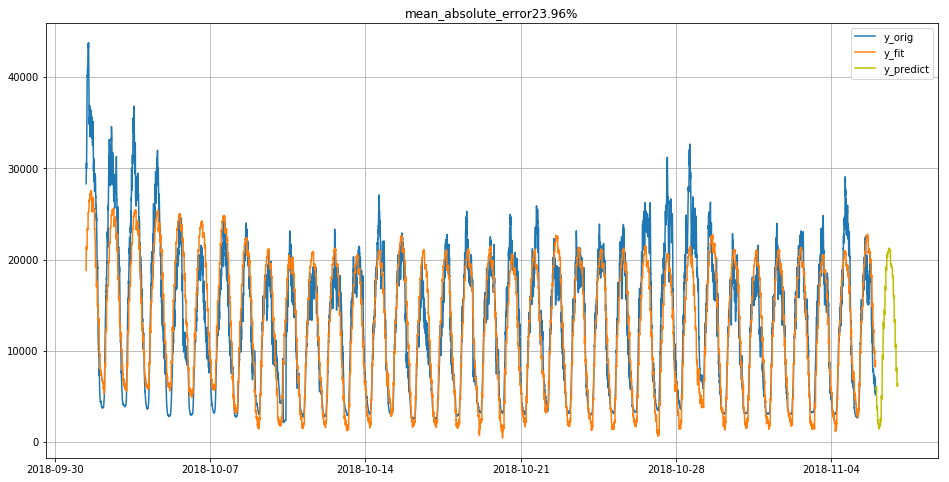特征重要性如下：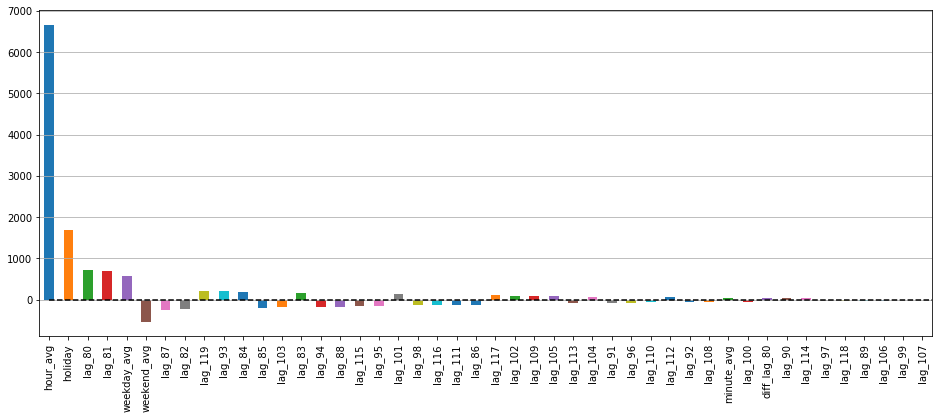介绍的比较简单，有不明白的，可以留言，探讨。
6.参考
主要参考了相关的博客，结合自己的数据，做了调整。
如果想学习，结合自己的业务，请见：
时间序列预测——深度好文中文
时间序列预测——ARIMA和随机森林对比英文
时间序列——各种方法分析英文
时间序列——预测方法对比中文
时间序列——Jason Brownlee博客系列推荐，有很多关于时间序列文章，但是英文的。


展开全文• 为了解决当下用电负荷预测精度不高，难以很好模拟实际用电负荷的分布情况而不能对未来的负荷数据进行合理预测的问题，实现了基于随机森林的分类模型、回归模型以及结合Weka的时间序列模型，对某省份的负荷数据进行...
• 工业过程中的数据挖掘对预测的不同机器学习模型的... 评估的模型类型是随机森林，朴素高斯贝叶斯，逻辑回归，K最近邻和支持向量机。 非基于时间的基于状态的方法与基于时间序列的方法的比较。 最终结果精度为99.83％
• prophet与基本线性模型（lm），一般加性模型（gam）和随机森林（randomForest）进行了比较。 首先，设置一些选项，加载一些库，并更改工作目录。 读取魁北克的出生文件，建立一个data.table 。创建培训和测试d.....数据模型 R语言 prophet
• 在解决上述问题时，本研究试图检验不同部门股票价格和 MV 之间的显着性程度，并使用随机森林 (RF) 和改进的留一法交叉验证预测 30 天的头部股票价格战术和长短期记忆循环神经网络 (LSTMRNN)。 与其他时间序列技术...
• 时间序列预测 不同时间序列预测模型的示例。 前处理 时间序列生成 正弦波和随机噪声的时间序列生成 具有趋势，季节性和随机噪声的时间序列生成 归因 外推法 相似 特征提取 TSFresh的时间序列功能 楷模 传统统计模型 ...
• 本文采用机器学习算法，如线性回归、随机森林等，完成时间序列预测预测效果也比较好。 2.数据集 本文对应的数据集格式如下： time value 2018-09-01 00:00 3221 2018-09-01 01:00 5515 2018-09-01 02:00 9971 … ...
• 根据随机森林（RF）预测的干扰概率的时间序列来检测干扰。 仅在Windows OS上测试了这些功能。 该过程需要很长时间才能映射大面积，并在并行计算中使用大量内存和CPU。 安装 您可以使用devtools从GitHub安装最新...R
• 随机森林模型选股matlab代码使用趋势确定性数据准备技术比较用于股票预测和股票指数走势的机器学习算法。 如果您喜欢演示文稿而不是自述文件，我们建议您查看该项目 :triangular_flag: 目录 :light_bulb: 介绍 2014...
• 值进行时间序列预测。 已将基于随机森林的算法用于确定性预测，并与持续性多云 (POC) 预测方法进行了比较。 支持向量回归 (SVR) 模型用于在概率预测的情况下预测每个时间戳的标准偏差值。 计算相应的误差指标，包括 ...
• 时间序列预测算法总结 文章目录时间序列预测算法总结前言一、...模型识别3 模型检验4 模型预测1.1.4时间序列分解模型1.2 基于机器学习的建模方法1.2.1 K近邻算法1.2.2 SVM1.2.1 随机森林1.2.2 Xgboost1.2.3 lightgbm机器学习 深度学习
• 时间序列分析 疫情模拟 机器学习与深度学习 明天 决策树与随机森林 无监督学习 关联规则 机器学习模型 股价预测 方法：自回归 loss SGD 线性回归（模型是什么样？损失函数是什么？）、逻辑回归 高斯分布，最大似然...线性回归 逻辑回归 聚类
• 从股票价格波动，到司机气温变化，从大桥沉降预测，到城市用电预警，时间序列数据广泛存在于量化交易、回归预测等机器学习应用，是最常见的数据类型。 接下来使用Python对一个经典的时间序列数据集：墨尔本十年气温...大数据
• 更具体地说，我们将使用均方根误差（RMSE）评估（i）随机森林，（ii）CNN单变量，（iii）CNN多变量，（iv）RNN-LSTM和（v）BiLSTM体系结构。 此外，我们将在以下两个维度上尝试不同的任务表述和框架类型：•我们将...
• 机器学习（XGBoost，套索，随机森林）：对时间序列数据进行单变量预测。 使用scikit-learn GridSearchCV函数可以完成超参数优化。 结论：套索表现更好！ 深度学习（TensorFlow，Keras）： ：使用的超参数优化。 这是...
• 补充：https://bmcbioinformatics.biomedcentral.com/articles/10.1186/1471-2105-15-276 如果用arima的话，还不如使用随机森林。。。 原文地址：...
• 使用随机森林机器学习算法对陆地卫星图像进行时间序列分析。 该算法将图像分为4类： 植被 城市的 裸土 水 为了进行分类，实施了不同的阶段。 第一阶段包括确定NDVI（归一化植被指数）和MNDWI（归一化差异水指数）...
• 股票价格预测是金融和学术研究中一个热门且重要的话题。 股票市场是一个不稳定的预测场所，因为没有重要的规则来... 尽管由于领域模糊，永远无法百分百准确地预测股票市场，但本文旨在证明随机森林预测股票价格的效率。
• 使用回归和时间序列建模技术预测每月产品的销售数量 特色技术： EDA 线性回归 森林随机回归 XGBoost 长短期记忆（人工循环神经网络） ARIMA时间序列预测 结果： 从XGBoost和LSTM模型获得了最佳结果 所有模型的...
• 除了标准最小二乘法、逐步法和Logistic法等传统的回归建模分析之外，JMP还提供了包括决策树、神经网络、时间序列随机森林、提升树、朴素贝叶斯等众多建模技术。 在数据挖掘方面，JMP既提供了探索性数据挖掘方法，...数据分析 大数据 数据挖掘 神经网络 机器学习
• 第30章 基于随机森林思想的组合分类器设计——乳腺癌诊断 第31章 思维进化算法优化BP神经网络——非线性函数拟合 第32章 小波神经网络的时间序列预测——短时交通流量预测 第33章 模糊神经网络的预测算法——嘉陵江...
• 本文为德国班贝克大学的硕士论文，共86页。 本论文是与西门子Healthineers合作撰写的。目标是预测X射线系统供应链的未来销售数字。...机器学习技术包括建模前馈神经网络和随机森林。所有方法的性能均.
• 与现有文献一致，我们发现，在时间序列设置中，来自 k 折的预测准确性估计存在乐观偏差，而消除数据“偷看”的交叉验证策略会产生较低且可能更现实的预测准确性估计. 更引人注目的是，我们还记录了概率的秩反转、...
• 我们将此问题视为回归问题，不采用时间序列方法，其中每个产品每个月（如果该月有销量）的相关信息作为一条记录，相关信息即为所提取的特征，包括产品固定信息和历史销量信息。对于无历史销量的产品，我们采用聚类的...